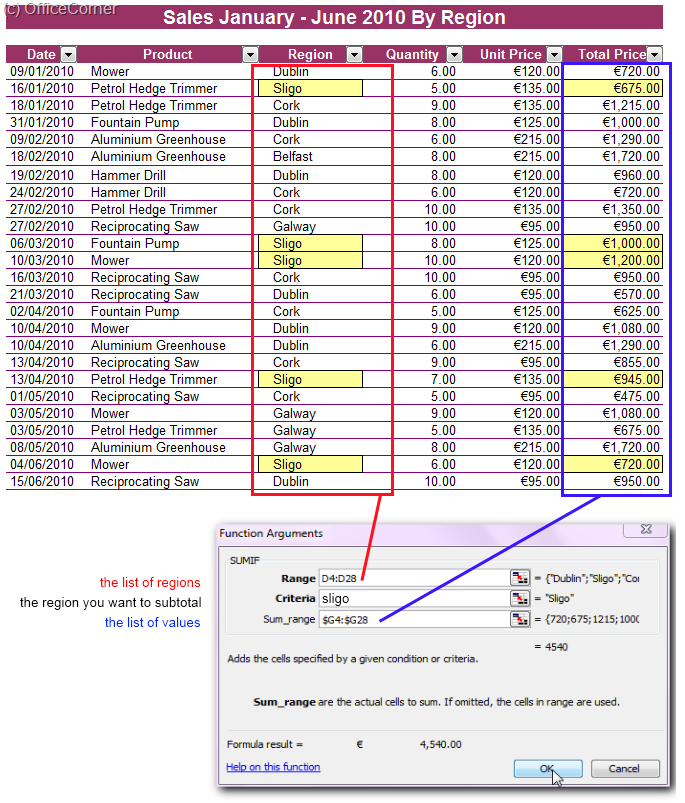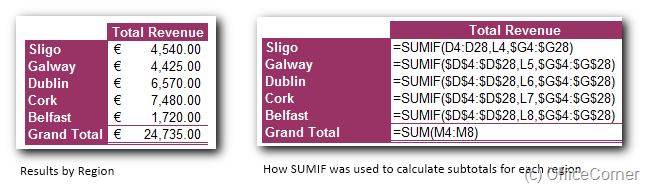Select Page

One advantage of using the SUMIF function to get subtotals is that it is not necessary to modify the order of the records in the original database unlike the Subtotals feature as shown in Super easy subtotals in a Microsoft Excel database.

## Using the SUMIF function to calculate the total by region

• The list of regions is in D4:D28 and is the first argument
• The Criteria is either the name of the region (Sligo) or a cell which contains the name (L4)
• The Sum_Range is the list of values and it must match the list of regions i.e. same height.## Using SUMIF in a Summary table

You can see in how the values calculated in the table of the left are obtained with the SUMIF function. Note that by using absolute references (\$D\$4:\$D\$28) it is easy to copy the formula and extend it to all rows at once with AutoFill.